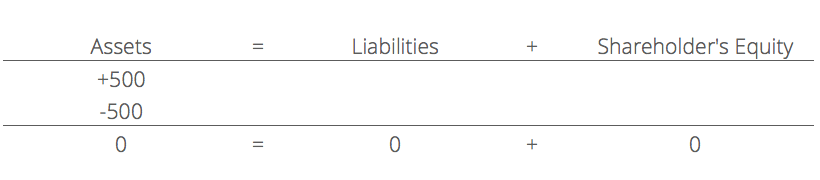# What is the Accounting Equation?

## Accounting Equation:

The accounting equation is the basic element of the balance sheet and the primary principle of accounting. It helps the company to prepare a balance sheet and see if the entire enterprise’s asset is equal to its liabilities and stockholder equity. It is the base of the double-entry accounting system.

Double-entry accounting is a system that ensures that accounting and transaction equation should be equal as it affects both sides. Any change in the asset account, there should be a change in related liability and stockholder’s equity account. While performing journal entries accounting equation should be kept in mind.

## How to calculate the Accounting Equation?

The accounting equation on the basis of a balance sheet can be calculated as.

• The business total assets should be located on the balance sheet for a particular period
• The liabilities of a company should be listed separately in the balance sheet and calculated
• The total liability and total stockholder’s equity should be added
• The total liabilities and equity will equal the company’s asset

Also Read: TS Grewal Solutions for Accounting Equation

## The Formula for the Accounting Equation

Assets = Liabilities + Shareholder’s Equity

## Example of Accounting Equation:

1. For the budgetary year, leading retailer ABC firm incorporated the following points on its balance sheet:

Total assets: ₹190 crore

• Total liabilities: ₹130 crore

Total shareholders’ equity: ₹60 crore

If we evaluate the accounting equation (Liabilities + Equity), we arrive at (₹130Cr + ₹50Cr) = ₹190 crore, which equals to the calculation of the assets submitted by the company.

2. An organisation ABC wish to buy a ₹500 manufacturing machine using cash. This deal will result in debt of (-₹500) for equipment and (+₹500) as a credit to cash. Therefore, the accounting equation will be.The above mentioned is the concept, that is elucidated in detail about ‘What is accounting equation?’ for the Commerce students. To know more, stay tuned to BYJU’S.

Must Read: DK Goel Solutions for Accounting Equation